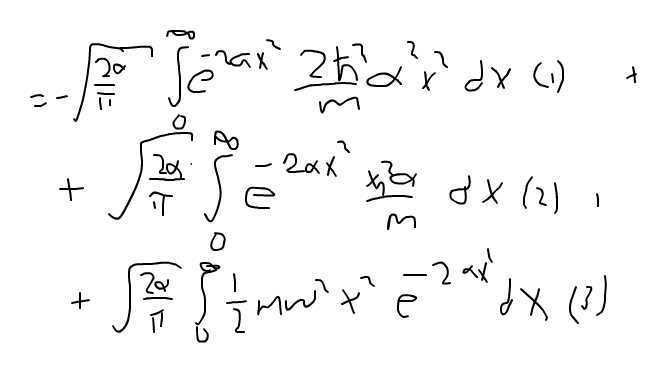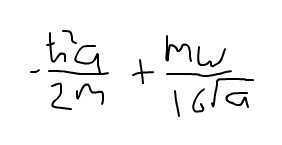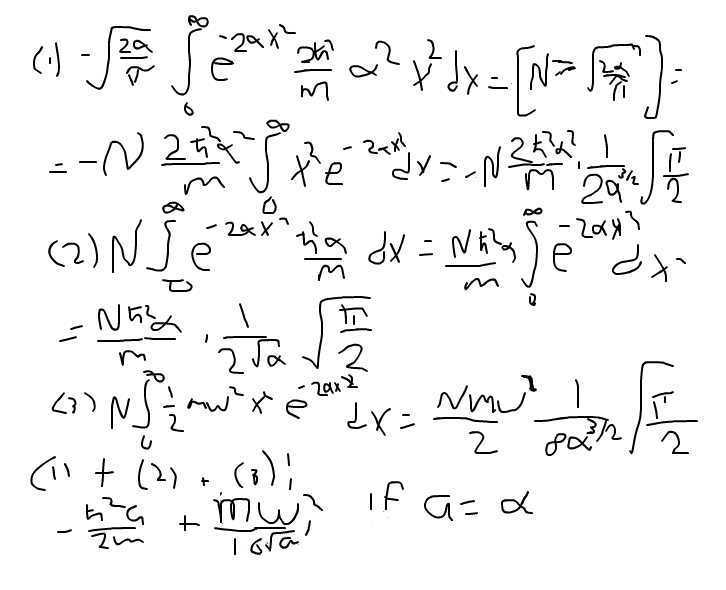# Variation method

## Homework Statement

Use the variation method to find a approximately value on the ground state energy at the one dimensional harmonic oscillator, H = -ħ^2/(2m) * d^2/dx^2 + 1/2mω^2*x^2

## Homework Equations

H = -ħ^2/(2m) * d^2/dx^2 + 1/2mω^2*x^2
u(x) = Nexp(-ax^2)
<H> = <u|Hu>

## The Attempt at a Solution

[/B]
Normilized N is N = (2a/π)^(1/4)

If i start to calculate Hu = N(-ħ^2/(2m)(4a^2*x^2 - 2a) + 1/2*m*ω^2*x^2)exp(-ax^2)

Then

<H> = ∫u*Hu dx = (2a/π)^(1/2)∫[0 to inf](-ħ^2/(2m)(4a^2*x^2 - 2a) + 1/2*m*ω^2*x^2)exp(-ax^2) dx
if i divide the integral into 3 different integrals i got the integral to be equal to -3ħ^2*a/(4m) + mω/(16*√a)) which is incorrect. It should be equal to ħ^2/(2m)*a + 1/8*m*ω^2*1/a why ?

## Answers and Replies

Is this the integral you mean?
$$\sqrt{\frac{2a}{\pi}}\int_0^\infty dx\left(\frac{-\hbar^2}{2m} (4a^2x^2-2a) + \frac{m\omega^2}{2}x^2\right) \exp{(-ax^2)}$$

The first thing I notice here is that you have forgotten the exponential from the complex conjugated wavefunction in your initial integral.
Secondly you need to show intermediate steps, we cannot see what has gone wrong. (not instantly at least)

In your next post you should mention what those 3 integrals you mentioned are. And how you solved them.
Solving should be quite straightforward as we are dealing with Gaussian integrals (we like those in physics).

A final piece of advise, try to use TeX to typeset your equations, its much more readable and accessible to potential helpers.
It's also useful for writing reports and papers if you need a lot of equations or diagrams.

Joris

I forgotted the complex term, but i used it when i calculated the integral through. See my steps in whiteboard bellow:
View attachment 85230
Here is the initial integral, <H>Here is im dividing the integral into 3 different parts (1), (2) & (3). When i have calculated the 3 different gaussian integrals and have add them together again, i got the following result:#### Attachments

So how did you calculate those separate integrals?

Explicitly, do you know the solutions of following integrals
$$\int_0^\infty x^2 e^{-\alpha x^2} dx$$
$$\int_0^\infty e^{-\alpha x^2} dx$$

What might help you here is introducing constants A, B and C for each factor in front of the integrals.
Afterwards you can simplify.

So now give me the values for those integrals as well as an expression for the result.

Edit:

I did a quick check of your result.
My result has an extra factor of 1/2 in each term. Are you positive the answer you gave in post #1 is correct?
It is possible I'm mistaken though as I only did a quick check.

Last edited:I still got the same result. I got the solutions for the integrals from the Mathematics Handbook for Science and Engineering

How did you get different results for the integral ##\int_0^\infty x^2e^{-2\alpha x^2}## in your first and third part?
That's where your mistake likely can be found.